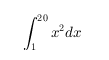# DataTipTemplate 属性

DataTipTemplate 属性控制数据提示的内容和外观。通过更改属性值，您可以修改数据提示的特定方面。

p = plot(1:10); dtt = p.DataTipTemplate

## 字体

• 'tex' - 使用 TeX 标记子集解释字符。

• 'latex' - 使用 LaTeX 标记解释字符。

• 'none' - 显示字面字符。

#### TeX 标记

^{ }上标'text^{superscript}'
_{ }下标'text_{subscript}'
\bf粗体'\bf text'
\it斜体'\it text'
\sl伪斜体（通常与斜体相同）'\sl text'
\rm常规字体'\rm text'
\fontname{specifier}字体名称 - 将 specifier 替换为字体系列的名称。您可以将此说明符与其他修饰符结合使用。'\fontname{Courier} text'
\fontsize{specifier}字体大小 - 将 specifier 替换为以磅为单位的数值标量值。'\fontsize{15} text'
\color{specifier}字体颜色 - 将 specifier 替换为以下颜色之一：redgreenyellowmagentablueblackwhitegraydarkGreenorangelightBlue'\color{magenta} text'
\color[rgb]{specifier}自定义字体颜色 - 将 specifier 替换为三元素 RGB 三元组。'\color[rgb]{0,0.5,0.5} text'

\alpha

α

\upsilon

υ

\sim

~

\angle

\phi

ϕ

\leq

\ast

*

\chi

χ

\infty

\beta

β

\psi

ψ

\clubsuit

\gamma

γ

\omega

ω

\diamondsuit

\delta

δ

\Gamma

Γ

\heartsuit

\epsilon

ϵ

\Delta

Δ

\spadesuit

\zeta

ζ

\Theta

Θ

\leftrightarrow

\eta

η

\Lambda

Λ

\leftarrow

\theta

θ

\Xi

Ξ

\Leftarrow

\vartheta

ϑ

\Pi

Π

\uparrow

\iota

ι

\Sigma

Σ

\rightarrow

\kappa

κ

\Upsilon

ϒ

\Rightarrow

\lambda

λ

\Phi

Φ

\downarrow

\mu

µ

\Psi

Ψ

\circ

º

\nu

ν

\Omega

Ω

\pm

±

\xi

ξ

\forall

\geq

\pi

π

\exists

\propto

\rho

ρ

\ni

\partial

\sigma

σ

\cong

\bullet

\varsigma

ς

\approx

\div

÷

\tau

τ

\Re

\neq

\equiv

\oplus

\aleph

\Im

\cup

\wp

\otimes

\subseteq

\oslash

\cap

\in

\supseteq

\supset

\lceil

\subset

\int

\cdot

·

\o

ο

\rfloor

\neg

¬

\nabla

\lfloor

\times

x

\ldots

...

\perp

\surd

\prime

´

\wedge

\varpi

ϖ

\0

\rceil

\rangle

\mid

|

\vee

\langle

\copyright

#### LaTeX 标记